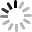# HTML5 控制裝置陀螺儀 ( 三軸 )## 起手式

``````window.addEventListener('deviceorientation', function(event) {
var alpha = event.alpha;
var beta = event.beta;
var gamma = event.gamma;
}, false);
``````

• aplha

行動裝置水平放置時，繞 Z 軸旋轉的角度，數值為 0 度到 360 度。• beta

行動裝置水平放置時，繞 X 軸旋轉的角度，數值為 -180 度到 180 度。• gamma

行動裝置水平放置時，繞 Z 軸旋轉的角度，數值為 -90 度到 90 度。## 實際測試

``````alpha:<span id="alpha"></span><br/>
beta:<span id="beta"></span><br/>
gamma:<span id="gamma"></span><br/>
``````

Javascript 的部分利用`window.addEventListener`監聽`deviceorientation`事件，接著就可以獲取 alpha、beta 和 gamma，不過獲取到的數值其實是個有非常多個小數點位數的數值，再利用 Math.round 來四捨五入。

``````if(window.DeviceOrientationEvent) {
var a = document.getElementById('alpha',
b = document.getElementById('beta'),
g = document.getElementById('gamma'),
alpha = event.alpha,
beta = event.beta,
gamma = event.gamma;

a.innerHTML = Math.round(alpha);
b.innerHTML = Math.round(beta);
g.innerHTML = Math.round(gamma);

}, false);
}else{
document.querySelector('body').innerHTML = '你的瀏覽器不支援喔';
}
``````## CSS 3D 的應用

HTML 的部分就放上 camera、space 和 box。

``````<div class="camera">
<div class="space">
<div class="box1">1</div>
<div class="box2">2</div>
<div class="box3">3</div>
<div class="box4">4</div>
<div class="box5">5</div>
<div class="box6">6</div>
</div>
</div>
``````

CSS 就比較複雜，不過因為不是這篇的重點所以就略過，有興趣的就去 玩轉 CSS 3D - 正四面體與正六面體 這篇閱讀，直接看到 js 的部分，沒有太特別，跟上一個範例幾乎相同，不過因為這裡我用了`document.querySelectorAll`，回傳的是一個陣列，所以要用`space`

``````var space = document.querySelectorAll('.space');
if(window.DeviceOrientationEvent) {

var alpha = event.alpha,
beta = event.beta,
gamma = event.gamma;

space.style.webkitTransform = 'rotateX('+beta+'deg) rotateY('+gamma+'deg) rotateZ('+alpha+'deg)';
space.style.transform = 'rotateX('+beta+'deg) rotateY('+gamma+'deg) rotateZ('+alpha+'deg)';
space.style.mozTransform = 'rotateX('+beta+'deg) rotateY('+gamma+'deg) rotateZ('+alpha+'deg)';

}, false);
}else{
document.querySelector('body').innerHTML = '你的瀏覽器不支援喔';
}
``````## 手機網頁指南針的應用

``````<div id="compass"></div>
``````

CSS 的寫法是這樣：

``````#compass{
margin:0 auto;
width:90%;
background-image:url(compass.jpg);
background-size:cover;
transform-origin:center center;
-moz-transform-origin:center center;
-webkit-transform-origin:center center;
}
``````

``````var compass = document.getElementById('compass'),
w = compass.offsetWidth;

compass.style.height = w +'px';

if(window.DeviceOrientationEvent) {

var alpha;
//     判斷是否為 iOS 裝置
compass.style.WebkitTransform = 'rotate(-' + alpha + 'deg)';
show.innerHTML = alpha;
}
else {
alpha = event.alpha;
webkitAlpha = alpha;
if(!window.chrome) {
webkitAlpha = alpha-270;
}
}

compass.style.Transform = 'rotate(' + alpha + 'deg)';
compass.style.WebkitTransform = 'rotate('+ webkitAlpha + 'deg)';
compass.style.MozTransform = 'rotate(-' + alpha + 'deg)';
}, false);
}else{
document.querySelector('body').innerHTML = '你的瀏覽器不支援喔';
}
``````